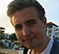# Summary of ROC Rules

This is a very short guide on how to find all possible outcomes of a system where Region of Convergence (ROC) and the original signal is not known.

### Summary of ROC Rules

1. For a causal system the ROC extends outwards.
2. For a non-causal system the ROC extends inwards.
3. For a two-sided system, the ROC can extend inwards or outwards from every pole.
4. The ROC cannot contain any poles
5. The system is stable if the unity circle is included in the ROC

### One Pole System Example

$$X(z) = \frac{1}{1-0.5z^{-1}}$$

1. Causal and Stable                                              2. Non-Causal and unstable

$x[n] = 0.5^nu[n]$                                                   $x[n] = -0.5^u[-n-1]$

Rule #1 and #5                                                        Rule #2$$X(z) = \frac{1}{1-2z^{-1}}$$

1. Causal and unstable                                          2. Non-Causal and stable

$x[n] = 2^nu[n]$                                                       $x[n] = -2^u[-n-1]$

Rule #1                                                                    Rule #2 and #5### Multiple Pole System Example

$$X(z) = \frac{1}{1-0.5z^{-1}} + \frac{1}{1-2z^{-1}}$$

This system has four possible ROC's, that is: there are four systems in the time domain that shares this z-transform.

1. Causal: $$x[n] = 0.5^nu[n] + 2^nu[n]$$

Rule #1 and #42. Non-causal $$x[n] = -0.5^nu[-n-1] - 2^nu[-n-1]$$

Rule #2 and #43. Two sided: $$x[n] = 0.5^nu[n] - 2^nu[-n-1]$$

Rule #3 and #54. Two sided: $$x[n] = -0.5^nu[-n-1] + 2^nu[n]$$

Rule #3[ - ]
Comment by November 25, 2015For someone like myself who is not familiar with ROC , what is ROC in layman's terms as it pertains to causal systems?
[ - ]
Comment by November 26, 2015Region of Convergence (ROC) is all possible values of z for which the Z transform converges. Pick any z outside of this region and it will diverge.

To post reply to a comment, click on the 'reply' button attached to each comment. To post a new comment (not a reply to a comment) check out the 'Write a Comment' tab at the top of the comments.